# Electric Conduction: solved equations

## Introduction

In an Electri c C onduction application the equations used for computation are:

• the corresponding Maxwell's equations for an electrical system, and
• the constitutive equation that characteriz es the conducting materials

The conditions of computation of an Electric Conduction application are the following:

• the computation concerns only the electric field intensity E; the fields D, B and H are not computed. The equations of the electric fields E, D and of the magnetic fields B, H are decoupled.
• the electric field is time independent (steady state): dE/dt =0

## Equations and conditions

In the previously defined conditions of computation, the equations are summarized as follows: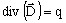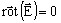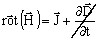⇒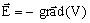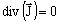E: electric field (in V/m) V: electric potential (in V) J: current density (in A/m 2 )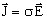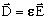⇒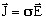σ : conductivity (in S)

The curl divergence of a field is always null: div [curl (Field)] = 0.

## Solved equation

The second order equation solved by the finite elements method in Flux in case of an Electric Conduction application is the following: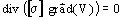where:

• σ is the tensor of the conductivity of the medium (in S)
• V is the electric potential (in V)

## State variable

The state variable in the Electric Conduction application is the electric potential V (written Ve in Flux 3D).

The uniqueness condition of the scalar field of the electric potential V requires that the value of this potential be assigned to at least one point of the computation domain.